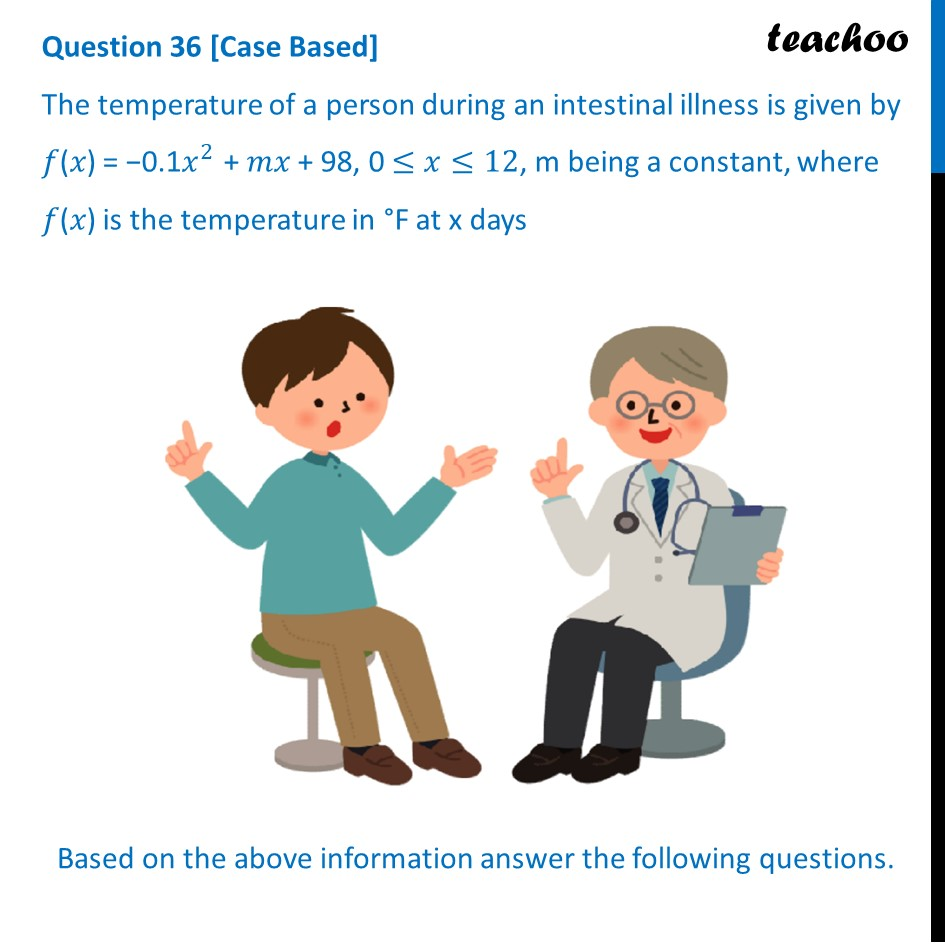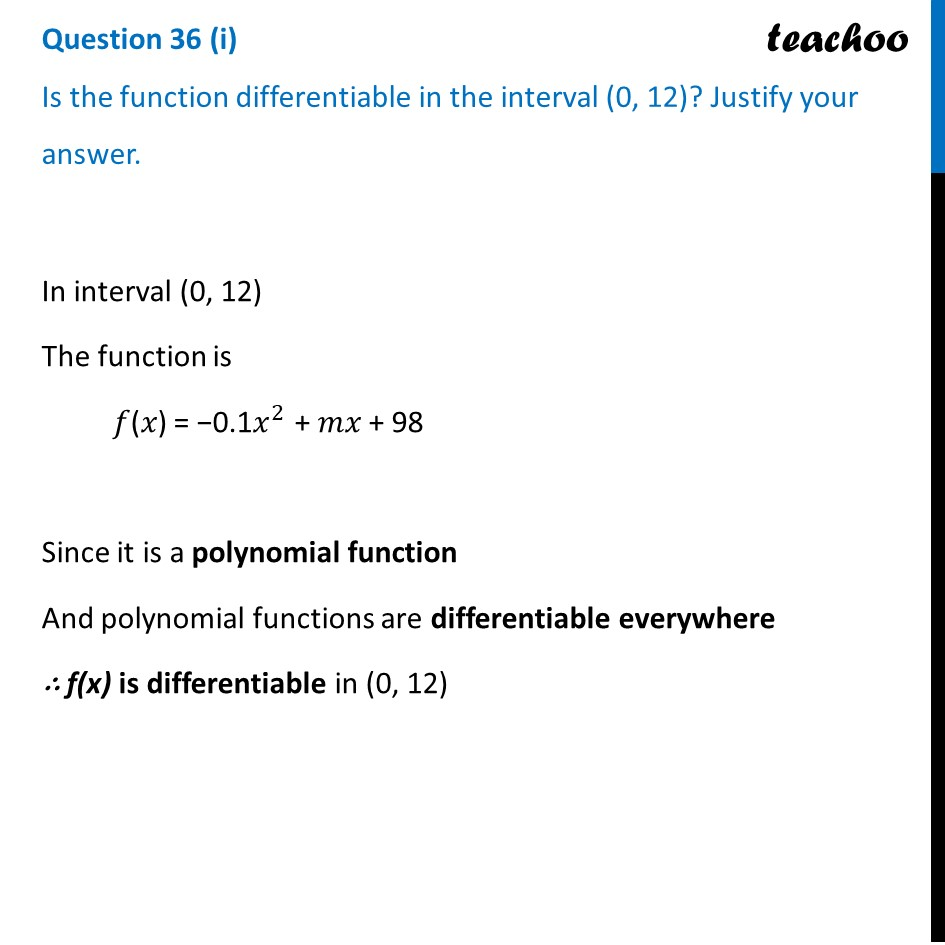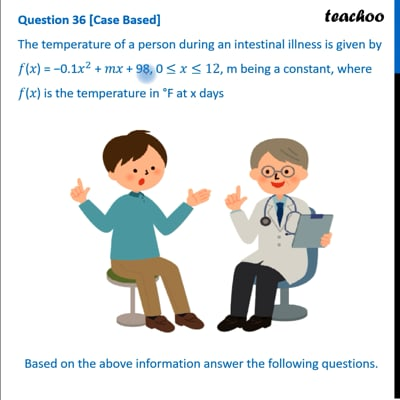CBSE Class 12 Sample Paper for 2023 Boards

Class 12
Solutions of Sample Papers and Past Year Papers - for Class 12 Boards

## The temperature of a person during an intestinal illness is given by 𝑓(𝑥) = −0.1x^2 + 𝑚𝑥 + 98, 0 ≤x≤12, m being a constant, where 𝑓(𝑥) is the temperature in °F at x days## Is the function differentiable in the interval (0, 12)? Justify your answer.This video is only available for Teachoo black users

Learn in your speed, with individual attention - Teachoo Maths 1-on-1 Class

### Transcript

Question 36 [Case Based] The temperature of a person during an intestinal illness is given by 𝑓(𝑥) = −0.1𝑥^2 + 𝑚𝑥 + 98, 0 ≤𝑥≤12, m being a constant, where 𝑓(𝑥) is the temperature in °F at x daysBased on the above information answer the following questions. Question 36 (i) Is the function differentiable in the interval (0, 12)? Justify your answer.In interval (0, 12) The function is 𝑓(𝑥) = −0.1𝑥^2 + 𝑚𝑥 + 98 Since it is a polynomial function And polynomial functions are differentiable everywhere ∴ f(x) is differentiable in (0, 12)